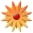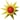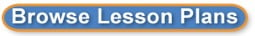# Graphing quadratic equations of the form f(x) = ax^2 + c

Page Views: 507

Email This Lesson Plan to Me
Overall Rating:(5.0 stars, 1 ratings)

 Keywords: graphing quadratic equations Subject(s): Grades 9 through 12 NETS-S Standard: Creativity and InnovationCommunication and CollaborationCritical Thinking, Problem Solving, and Decision MakingView Full Text of Standards School: Duncan Polytechnical High Sch, Fresno, CA Planned By: Pineda Erick Original Author: Pineda Erick, Fresno
Date:04/06/2018 Grade Level: 9 Unit/Lesson Topic: Algebra 1 Lesson 8.2  Day 1: Graphing f(x) = ax^2 + c
Essential Question: How does the value of c affect the graph of f(x) = ax^2 + c?

Content Standard(s): HSA-CED.A.2 HSF-IF.C.7a HSF-BF.B.3

ELD Standard(s): ELD.PI.2, ELD.PI.3, ELD.PI.5, ELD.PI.7, ELD.PI.10

Content Objective(s)
What are learners going to learn

Students will graph quadratic functions of the form f(x) = ax^2 + c. Students will solve real-life problems involving functions of the form f(x) = ax^2 + c. Previous Learning: Students have a prior understanding of vertical translations Previous Vocabulary: translation, vertex of a parabola, axis of symmetry, vertical stretch, vertical shrink, and reflection.

Language Objective(s)
How are learners going to learn

Students will use new vocabulary by repeating the new words and associating them with examples. They will explain solution to partner(s), and to the class-projector and speaking answer.
Students, when showing answer, will use such vocabulary as Parabola shifted down/up, projection.
Students will associate the meaning of shift with movement up or down and will practice with examples on their student journal.
Students will practice previous vocabulary by using it again in this section-vertical stretch or vertical shrink.

Features of Integrated ELD
&#61660; Interactive and Engaging
&#61660; Focus on Content Knowledge
&#61660; Clear Lesson Objectives
&#61660; Corrective Feedback
&#61660; Formative Assessment Practice

Key Vocabulary:

Materials: Document camera or overhead projector, Student Journal-Big Ideas, chrome books, paper, pencil.

Procedures/Instructions:
 Post/Share Objectives and Expectations
o Language Frames
o Rubrics
 Anticipatory Set
o Activate knowledge
o Build Background
o Create Interest
 Direct Instruction
o Collaborative Structures
o Comprehension/ Meaning Making Strategies
o Instructional Strategies
o Critical Thinking Skills
o Language Forms & Functions Skills
 Guided Practice
o Collaborative Structures
o Formative Assessment/Feedback
 Independent Practice
o Scaffolds
o Collaborative Structures
 Closure
o Revisit Objectives
o Checks for Understanding

Warm Up (6 minutes)

Review previously assigned homework, if necessary, and review vocabulary by asking random students to read words on the poster boards and white board
.

Before Start Thinking question from Big Ideas, teacher explains that todays objective is to observe how the value of C-a constant-affects the quadratic function ax^2 + c.

Teacher projects Start Thinking Question (ELD.PI.3) (5 minutes):

Use your knowledge of the of the graph of y = ax^2 to describe the effect of the constant c in the equation y = ax^2 + c. Describe the difference in the appearance of the graph of f(x) = ax^2 and f(x) = ax^2 + c.

Warm Up (6 minutes)

Review previously assigned homework, if necessary, and review vocabulary by asking random students to read words on the poster boards and white board
.

Before Start Thinking question from Big Ideas, teacher explains that todays objective is to observe how the value of C-a constant-affects the quadratic function ax^2 + c.

Teacher projects Start Thinking Question (ELD.PI.3) (5 minutes):

Students give different answers (ELD.PI.7, ELD.PI.3), and teacher guides conversation by asking questions.

After Start Thinking question and after students have given answers to the posed question, teacher discusses how C actually affects the quadratic function (what type of shifting C has on function).

Core Concept  Graphing f(x) = ax^2 + c (5 minutes)

In this Core Concept, students learn how to graph f(x) = ax^2 + c. Teacher writes the Core Concept, relates it to vertical translations of linear functions, and uses different colors to connect the equations and the graphs.

Focus on Mathematical Practice 1 (ELD.PI.2, ELD.PI.3, ELD.PI.5)

Teacher writes two examples on the board (g(x) = x^2 + 5 and x^2 - 5). Teacher asks students to talk to each other and find out what happens on each graph but no graphing is happening. Teacher asks for some answers randomly and then explains the effect of c on both graphs.

Teacher gives new examples and lets students work in pairs to solve the functions (ELD.PI.3, ELD.PI.5).
f(x) = x^2
f(x)= x^2 + 3
Teacher walks around to hear students use correct vocabulary-square negative 2 and add three; the Parabola shifts up by three.
Teacher lets some students share answers with the whole class by projecting it on the board (ELD.PI.2).

Note:
Students should be comfortable with graphing y = x^2 and y = x^2 + 3 and explaining the effect of the constant a (previous lesson) on the graph of y = ax^2. Then, they begin to make sense of another parameter when they graph y = ax^2 + c. Using tabular and graphical representations, they make sense of the effect of c.

EXPLORATION 1 (Student Journal) (ELD.PI.2, ELD.PI.3, ELD.PI.7) (8 minutes)

Graphing y = ax^2 + c

In this exploration, students sketch the graphs of quadratic functions that have been vertically translated.
Have students work in pairs to complete parts (a) and (b).
Students should be comfortable graphing functions of the form y = ax^2 by making a table of values, plotting the ordered pairs, and drawing a smooth curve (parabola) through the points. Students add the constant C to y = ax^2 and discover that such constant shifts parabola up by C units.
Students are graphing a pair of functions in the same coordinate plane.
Students discuss the characteristics of each graph, such as parabola opens up or opens down, wider or narrower-thinner-than y = x^2,and shifts up or down.
Students may only notice that the vertex is no longer at (0, 0). They may not think of c as a vertical shift.
Teacher paces room¸ observes, and guides students for support.
Students share answers with whole class by using the projector.

In this next exercise, students graph vertically translated quadratic functions and compare it to the graph of the parent quadratic function. Have students use Think-Pair-Share to complete the handout (ELD.PI.2, ELD.PI.3, ELD.PI.10). (12 minutes)
Students are given a handout with three quadratic functions, which they will graph.
Teacher explains the rules for graphing all functions.
Students graph three functions individually (no help from partner nor teacher).
Students compare answers with partners and decide which function should be chosen for the correct answer to be placed on handout (The answer chosen to be placed on handout has been carefully checked by both students).
Students use Desmos to graph all three functions and compare them to their own answers and the answers chosen by both students.
Student and partner find their mistakes, if any, and prepare to share findings with the whole class.
Teacher paces the classroom throughout activity and randomly asks specific questions to some students to make sure students use correct academic language.
Teacher checks for understanding by asking for volunteers to share their graphs and explain how they found answers (Teacher chooses on incorrect answer to give opportunity for discussion)
Teacher finishes lesson by making all students recite vocabulary words, which are written on the whiteboard.

Assessment (Content and Language):
 Formative
 Summative
 Homework
 Projects

ASSESS(3 minutes)

Closure (as time permits)

Exit Ticket (ELD.PI.7): Describe the effect of C on the graph of f(x) = x^2 + C.
Homework Assignment (Difficulty Level-high, medium, and low): Student Journal: pages 253 and 254.

 Materials: Whiteboards, Video Cameras, Power, High, ESL